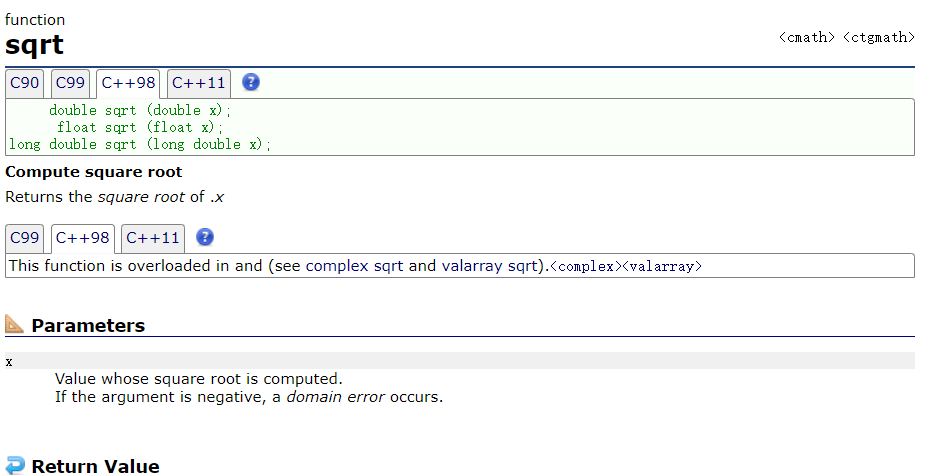6 篇文章 0 订阅

# 什么是素数

## 如何计算素数

1)：因此判断一个整数num是否为素数，只需用 2 ~ num-1 （因为所有整数都能被1整除）之间的每一个整数去除num，如果都不能被整除，那么 num即一个素数。

//如判断数num是否为素数
flag = 0;
for(int i=2;i<num;i++)
{
if(num%i==0)
flag = 1;
}
if{flag == 0}
{ //是素数 }
else
{ //不是素数 }


2)：num不必被 2 ~ num-1 之间的每一个整数去除，只需被 2 ~ √num之间的每一个整数去除就可以了。如果 num不能被 2 ~ √num之间任一整数整除，num 一定是素数。

// An highlighted block
flag = 0;
for(int i=2;i<=sqrt(num);i++)
{
if(num%i==0)
flag = 1;
}
if{flag == 0}
{ //是素数 }
else
{ //不是素数 }### 素数源代码

（1）素数判断

// **判断num是否为素数**
#include<stdio.h>
#include<windows.h>
#include <math.h>
#pragma warning(disable:4996)   //vs（vs2013）使用scanf时需要加 _s 否则会报错，用这种是解决方法之一

int main()
{
int num, i;
int flag=0;              //判断标志
scanf("%d", &num);  //输入要判断的整数num
if (num == 0){
flag = 1;
}
for (i = 2; i <= sqrt(num); i++)
{
if (num%i == 0){
flag = 1;
break;
}
}
if (flag == 0){
printf("%d是素数。\n",num);
}
else{
printf("%d不是素数。\n", num);
}
system("pause");   //vs运行会直接结束运行窗口自动关闭，可以用<window.h>中的syetem函数使运行窗口保留。
return 0;
}



（2）输出1~100所有的素数

// **找出1~100中所有素数**
#include<stdio.h>
#include<Windows.h>
#include <math.h>

int main()
{
int i = 2,flag=0;
for (; i <= 100; i++)
{
flag = 0;
int j = 2;
for (; j <=sqrt(i); j++){
if (i % j == 0){
flag++;
break;
}
}
if (flag == 0){
printf("%d  ", i);
}

}
printf("是素数。\n");
system("pause");   //vs运行会直接结束运行窗口自动关闭，可以用<window.h>中的system函数使运行窗口保留。
return 0;
}



05-11
10-1603-132万+
08-133万+
05-2046
12-041280
01-04262
09-1692
07-19971
02-17
05-032947
03-18193
01-25373
04-062万+
12-201727
11-24487点击重新获取扫码支付余额充值